## Phases of a tsunami

### Generation

A tsunami often results from the sudden change in the topography of the ocean e.g. a seism due to plate tectonics or a landslide. But it can also be created by a meteorite impact.The relationship between the height of the tsunami wave and the magnitude of a seism is complex. It strongly depends on the relative displacements (velocity, acceleration,…) of the plates during the earthquake, a large but slow displacement will create a small wave. Moreover the local topography of the coast (strong slopes of the seabed) can significantly modify the wave height (resonance effects).

Click on thumbnail to watch### Propagation

Once the tsunami is triggered, we enter the phase of propagation. Contrary to what one might think, the wave of tsunami in the ocean is not visible. Indeed, his height does not exceed 50 cm and the wave extends over 100 km! A boat would not notice, therefore, it was long thought that the tsunamis were forming near the coast. Sailors left on the high seas didn’t see the tsunami until they they returned to the port and everything was a shambles! On the other hand, if the tsunami remains little visible on the high seas, there moves very fast, up to 800 km/h (almost the speed of sound!). Thus, the evacuation is almost impossible for tsunamis declaring themselves too close to the sides.

Click on thumbnail to watch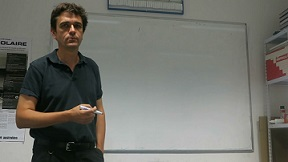### Surge

Approaching on sides, the tsunami slows down, and at the same time its height increases up to claim a few meters. Specific geographies can increase this height up to 60 m. Generally, the tsunami wave is not significantly larger than an ordinary wave, but unlike her, tsunami wave carries astronomical amounts of water, and can therefore penetrate the Earth and wreak havoc.

Click on thumbnail to watch## Modelisation/Simulation

This video is the result of a calculation made on a computer, a digital simulation and not the return of satellite images. Color gives the elevation of the surface of the ocean (in red an elevation). How do we get such images? We must first build a mathematical model that describes the phenomenon, then use an algorithm.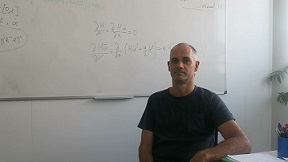Interview with Jacques Sainte-Marie, reasercher at INRIA Paris.

### Modelisation

The modelisation of a tsunami isn't simple. The tsunami is a physical phenomenon described by the fluid mechanics and revealed complicated equations. These equations are called "partial differential equations.". A tsunami is composed of three phases: initialization, propagation and surge. The scales are very different for the three phenomena, it is hardly possible to have a global vision of the phenomenon. The solutions of the equations are functions of several variables (time and space). These equations of the fluid mechanics are based on simple principles: conservation of mass, conservation of energy, second law of Newton (acceleration proportional to the sum of the forces applied to the system). However, the partial differential equations of the fluid mechanics are impossible to solve analytically. Therefore, we have to use numerical methods. The goal is then to reduce the cost of calculations, using simpler models. One of the most used for the propagation of tsunamis is the shallow water equations. This may seem counter-intuitive: in fact we tend to say that a flow in an ocean is necessarily deep. We must actually compare characteristic quantities: for example the depth of the ocean and the wavelength of the tsunami (distance between two waves). A tsunami can have a wavelength exceeding 100 km. In an ocean with a depth of 4000 m for example, the report of the two lengths gives 0.04, the hypothesis of shallow water is valid. By making this approximation and using for example the shallow water model, we find that the speed of the tsunami depends mainly on the acceleration of gravity g (about 10 m/s 2), and the water depth h.

Precisely, the speed of the tsunami is the square root of the height multiplied by the acceleration of gravity. That's why a tsunami spread very fast off the coast of the ocean and slows down considerably when it gets closer beaches.

Choose the depth oh the ocean (m)

### Numeric simulation

Once the mathematical model has been built, we can move on to numeric simulation. These simulations are very important. First of all by a beam of simulations, We can know what scenarios are likely to occur and modify the infrastructure accordingly. In addition, simulations also provide a better understanding of the physical phenomena. A numeric simulation is to make calculations with a computer through the mathematical model thanks to an algorithm. There are several steps to simulate a tsunami. We must first define the seabed and the initial conditions due to the earthquake. Then, we have to discretize the problem to allow the computer to make calculations, i.e. cut the region of space where the tsunami spread and define a (small) step of time. For the simulation to be viable, several conditions must be verified. First, the convergence: when cells in space and time step become increasingly small. The numeric solution has to converge to the analytic solution. However, the compution time must be reasonnable. If cells in space and time step are very small, the computer must do a lot of calculations, and computing time is long.

## Historical tsunamis

This map contains simulations and facts about seven tsunami-catastrophes. Find them, by clicking on it’s potential location!

Map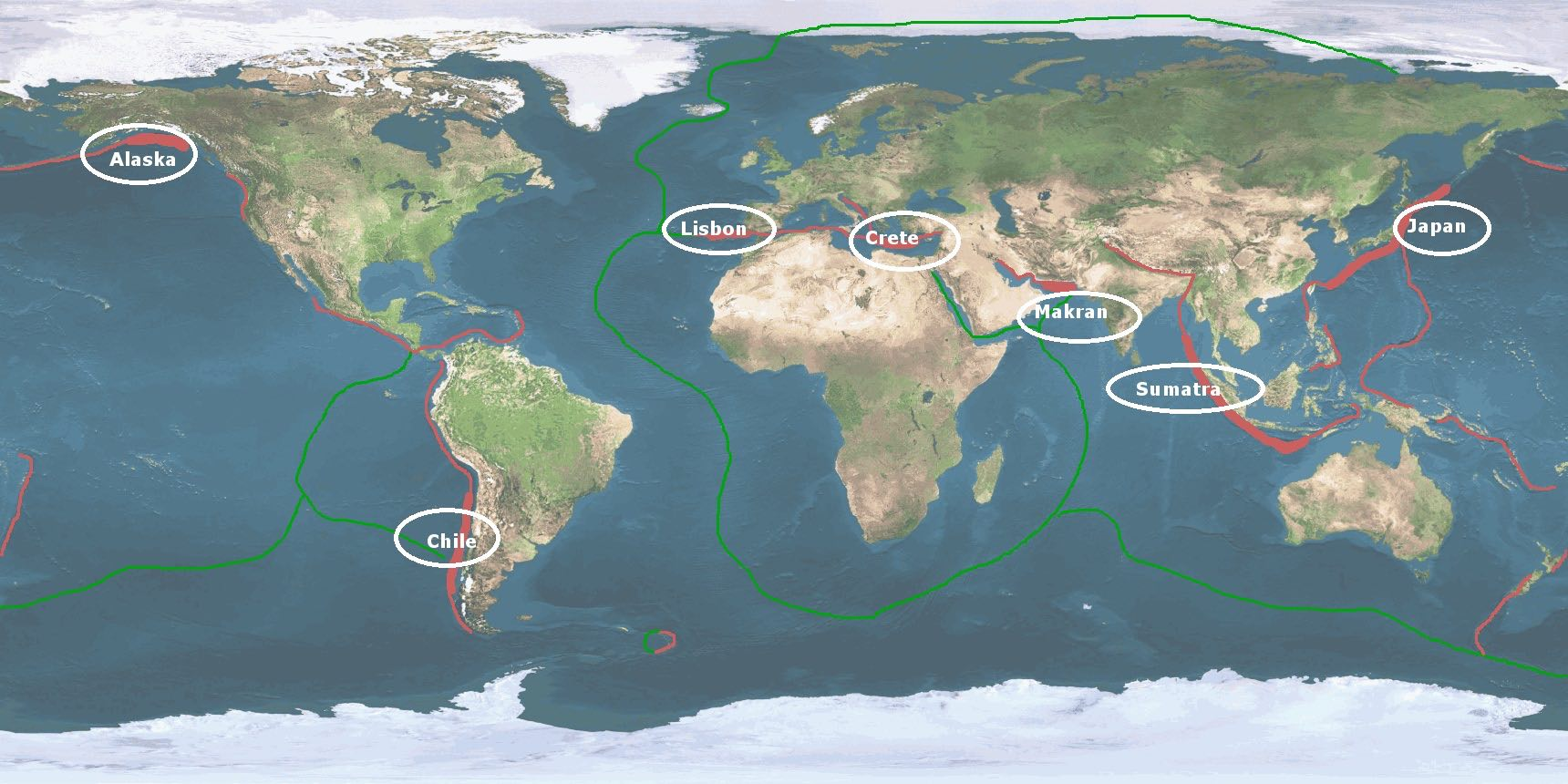## validation/applications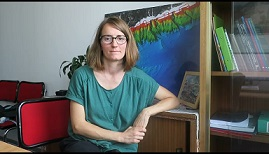Click on thumbnail to watch an interview of Sara Lafuerza, researcher at University Pierre et Marie Curie.Click on thumbnail to watch an interview of Anne Mangeney, researcher at Institut de physique du Globe de Paris.

Like seisms, tsunamis are difficult to predict. Buoys located in deep ocean recording the sea levels variations can detect the propagation of a tsunami and trigger early warning systems. Another approach to reduce the potential impact of tsunamis is to build realistic scenarios of seisms, to calculate the induced wave propagation and its arrival on the coasts. Then modifications of infrastructures can be planned.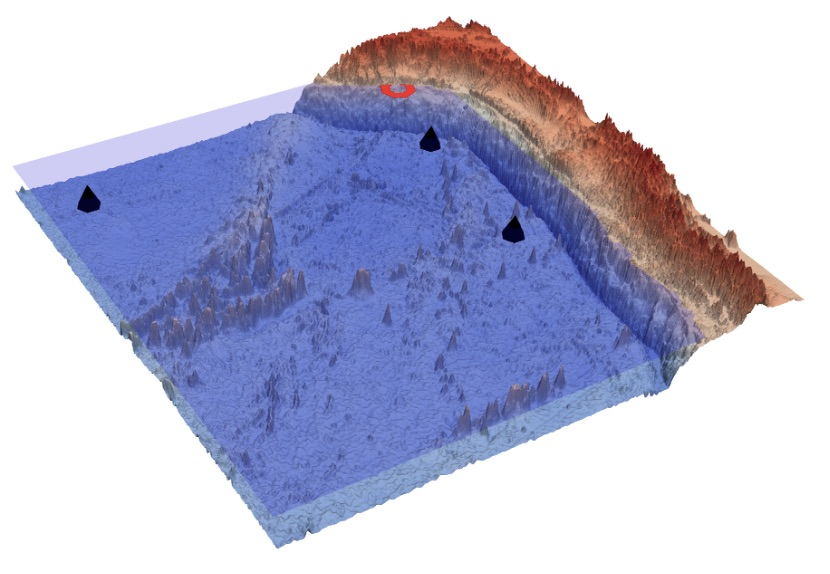An exemple of buoys (black points) located on the Chilean coast.